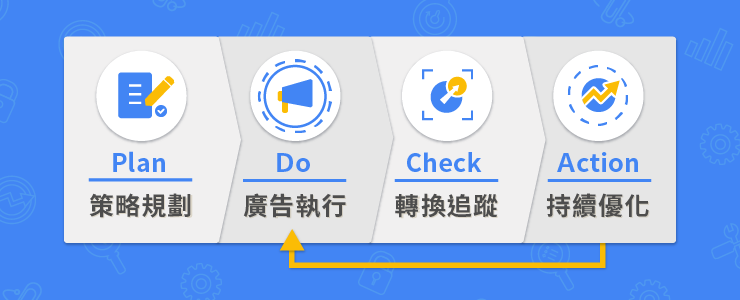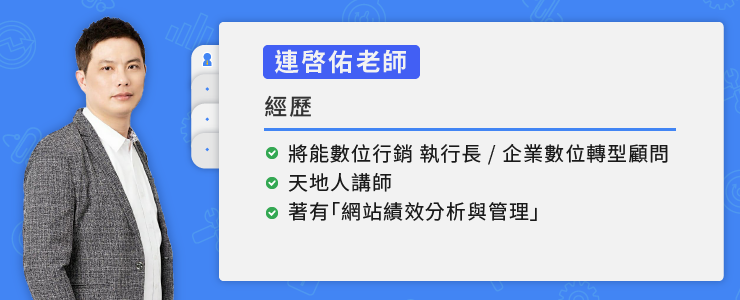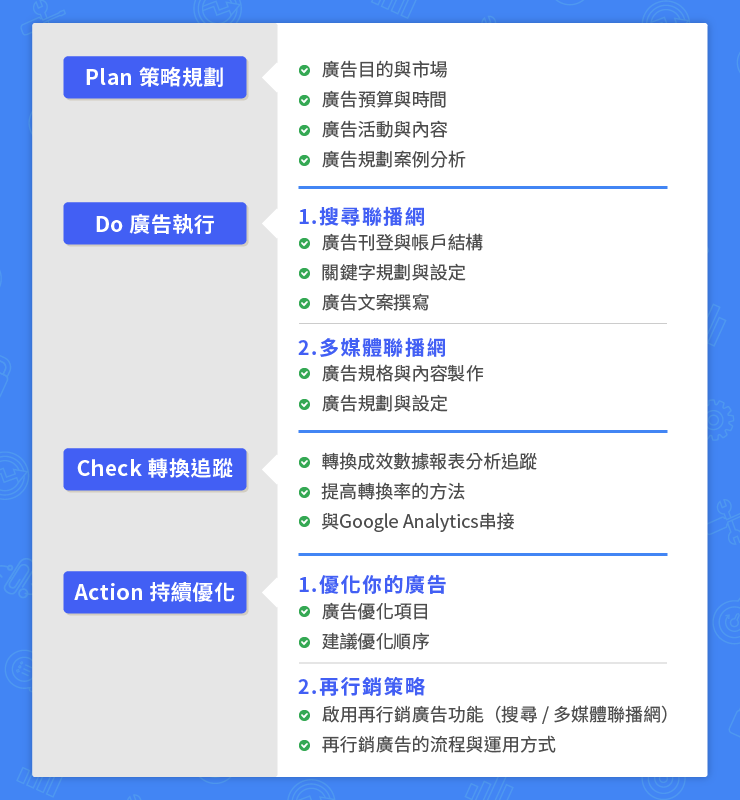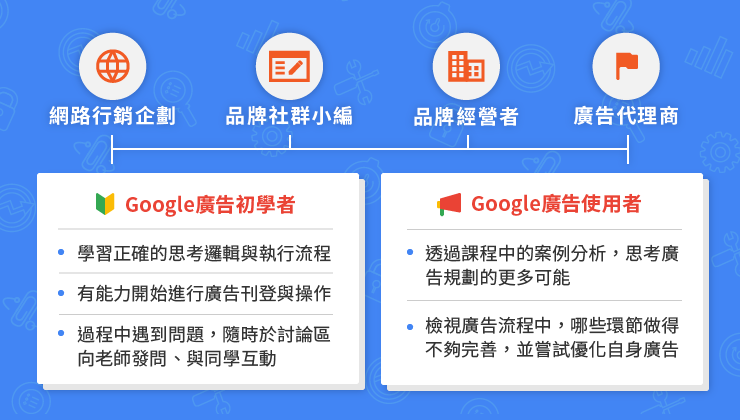2

8分鐘

1

• Plan 規劃
• 1 確認目標受眾與主要市場 03:51
• Check 追蹤
• 2 轉換追蹤 04:11

### 開始分享你的學習成果吧！

<%if list.length == 0 %><%else%> <%props list%>

<%if prop.type == 'image' %>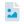圖片檔 <%else prop.type == 'pdf' %>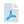PDF <%else prop.type == 'youtube' %>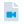Youtube 影片連結 <%else prop.type == 'ppt' %>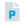PowerPoint <%else prop.type == 'sound' %>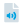錄音檔 <%/if %>

<%:prop.title%>

<%/props%> <%/if%>

### 作業觀摩<%props data%>

<%if prop.type == 'image' %><%else prop.type == 'pdf' %><%else prop.type == 'youtube' %><%else prop.type == 'ppt' %><%else prop.type == 'sound' %><%/if %> 作業<%>~numToZH(~toInt(key) + 1)%> <%:prop.title%>

<%/props%>
<%props data%>
<%if prop.type == 'image'%><%if prop.cover %>
<%:~isGif(prop.cover)%>
<%else%>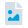<%/if%><%else prop.type == 'pdf' %>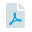<%else prop.type == 'ppt' %>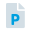<%else prop.type == 'sound' %>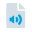<%/if%>
<%if prop.visible == 1 %><%/if %>

<%:~formatDate(prop.create, 'YYYY-MM-DD')%>

<%:prop.title%>

<%if prop.isAuthor == true %>刪除編輯<%/if %>

<%:prop.comment_count%>

<%/props%>

# 來關注最新的課程資訊吧！<%props data%>

<%:~formatDate(prop.publishDate, 'YYYY/MM/DD')%>

<%:prop.title%>

<%if ~root.isStudent === false && ~root.isTeacher === false && prop.permission === 'private' %>

<%else%> <%:prop.content%> <%/if%>
<%/props%>